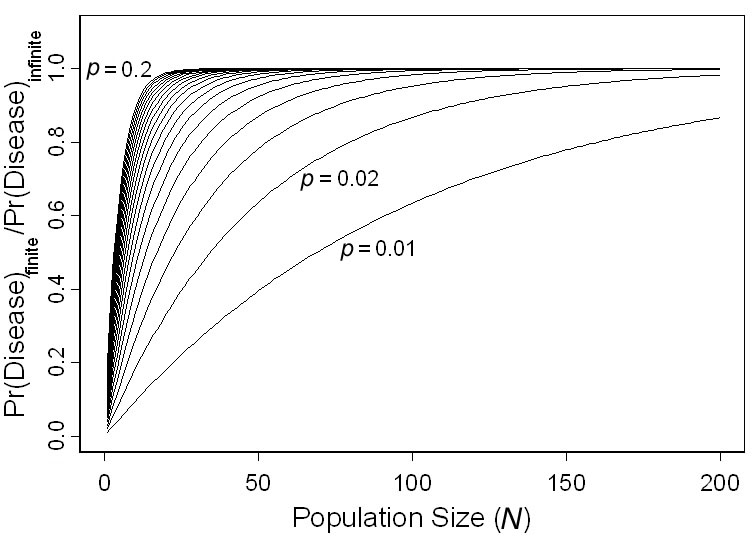### Michael J. Adams, Nathan D. Chelgren, David Reinitz, Rebecca A. Cole, Lara J. Rachowicz, Stephanie Galvan, Brome McCreary, Christopher A. Pearl, Larissa L. Bailey, Jamie Bettaso, Evelyn L. Bull, and Matthias Leu. 2010. Using occupancy models to understand the distribution and prevalence of an amphibian pathogen, Batrachochytrium dendrobatidis. Ecological Applications 20:289–302.

Appendix A. A figure showing ratio of the probability of disease occurrence in a finite population ψ[1-(1-p')N] to the probability of disease occurrence in an infinite population, ψ.FIG. A1. Ratio of the probability of disease occurrence in a finite population ψ[1-(1-p')N] to the probability of disease occurrence in an infinite population, ψ. The ratio approaches 1 as finite population size (N) increases and detectability (p) increases.

[Back to A020-006]